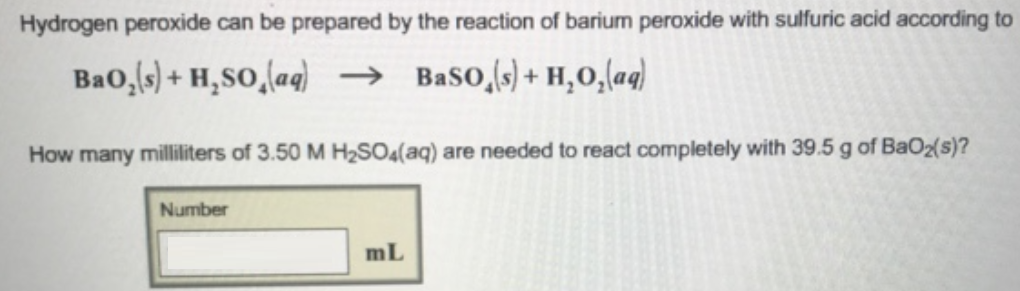# Problem: Hydrogen peroxide can be prepared by the reaction of barium peroxide with sulfuric acid according to BaO2 (s) + H2SO4 (aq) → BaSO4 (s) + H2O2 (aq) How many milliliters of 3.50 M H2SO4(aq) are needed to react completely with 39.5 g of BaO2 (s)? Use the periodic table to determine the molar mass of BaO2.

###### FREE Expert Solution
87% (244 ratings)###### Problem Details

Hydrogen peroxide can be prepared by the reaction of barium peroxide with sulfuric acid according to

BaO2 (s) + H2SO4 (aq) → BaSO4 (s) + H2O2 (aq)

How many milliliters of 3.50 M H2SO4(aq) are needed to react completely with 39.5 g of BaO(s)? Use the periodic table to determine the molar mass of BaO2.